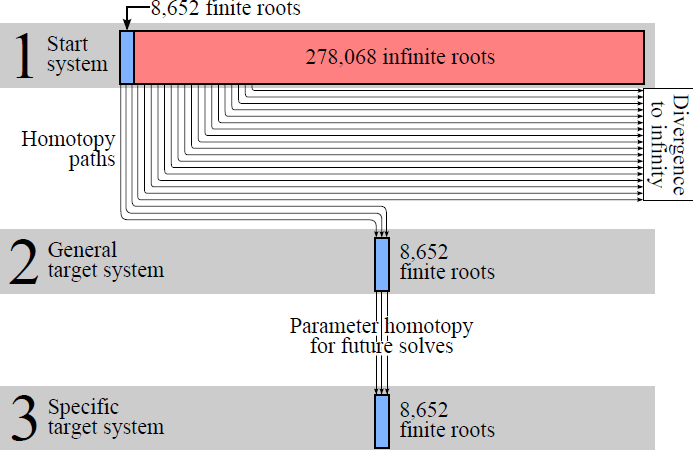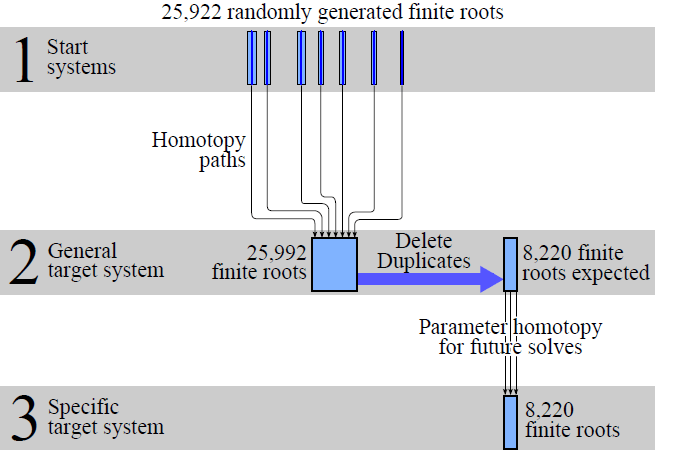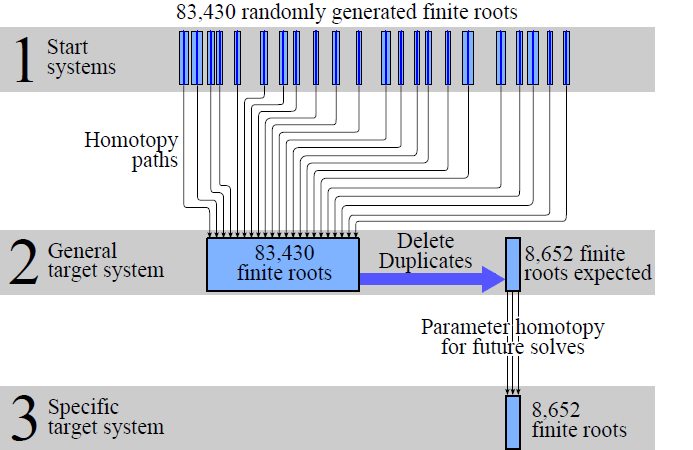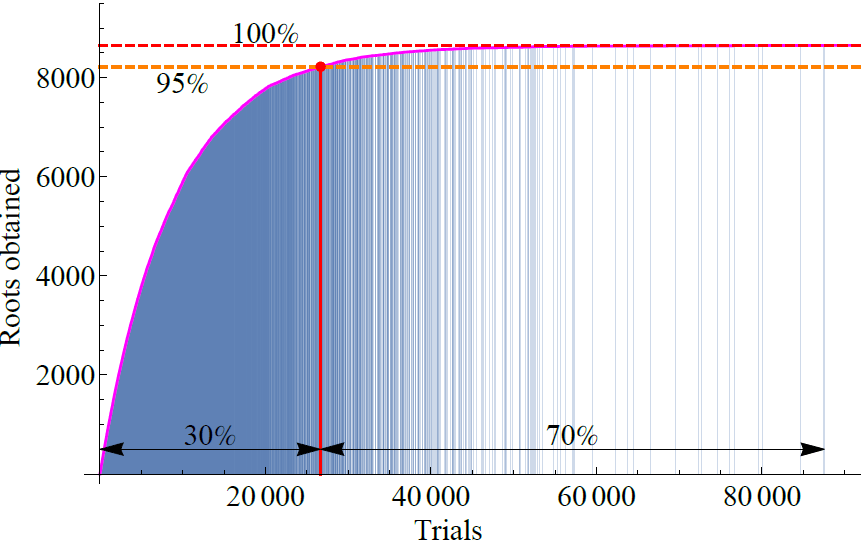Mark Plecnik, PhD

Discovery and Invention through Computational Mechanical Design

## Finite Root Generation

Finite Root Generation (FRG) is a method to collect all roots of a polynomial system.  Particularly, FRG is useful for solving large systems of equations associated with mechanism synthesis problems.  It uses homotopy continuation to accomplish this goal.

Homotopy continuation finds all roots (endpoints) of a target system by first constructing a start system from which all roots (startpoints) are readily obtainable.  Then the start system is deformed into the target system and each startpoint is tracked to an endpoint, transforming the task of algebraic root finding into solving several independent ordinary differential equations.

In the case of multihomogeneous homotopy, each equation of the start system is a product of linear expressions.  In this way, each startpoint is generated by setting one linear expression from each equation equal to zero and solving the resulting linear system.  Constructing all startpoints involves exhaustively going through these combinations, leading to characteristic explosion.

For the synthesis systems we are interested, the large majority of these startpoints track to endpoints at infinity.  This is due to a degeneration in monomial structure from start system to target system, and these infinite roots serve no purpose but to be filtered out.  Consider the four-bar nine point path generator problem below.

### Multihomogeneous homotopy:(1) The combinatorics generate 286,720 startpoints, of which 97% track to roots at infinity.  (2) Once the set of 8,652 finite roots is found, this data may be used again and again to find finite root sets of other systems with the same monomial structure (3).  This is the standard approach in homotopy continuation: splitting the problem into a computationally expensive ab initio solve followed by cheaper reproduction from the resulting data.

The goal of FRG is to avoid tracking all roots at infinity.  The method accomplishes this, but with a trade-off: startpoints are guaranteed to track to finite roots, but are generated using a stochastic process that may track to the same nonsingular endpoint more than once.  Before getting into the algorithm, let’s examine the implications of this process.

For our benchmark problem, let’s assume that with each iteration of FRG, the algorithm might happen upon one of any of the 8,652 finite roots with equal probability.  In the beginning, the algorithm would always be finding new roots, as the chances of randomly finding one of the 8,652 roots multiple times in a small number of iterations is quite low.  But as more and more roots are collected, the likelihood of finding a previously collected root increases.

This rate of duplication is modeled nicely by a classical problem in probability theory: the coupon collector problem.  This allows us to model the expected relationship between FRG iterations and the number of unique roots collected.  We apply this model to the benchmark problem.

### Collecting 95% of the finite root set:(1) Only 25,922 startpoints need to be constructed to find 95% of the finite root set, that is 91% less homotopy paths to track compared to multihomogeneous homotopy.  The resulting finite roots (2), although incomplete, can be used to find 95% of finite roots for other systems (3) with the same monomial structure.

### Collecting 100% of the finite root set:Repeating this exercise for finding 100% of roots indicates a situation of diminishing returns: 83,430 paths must tracked to find all roots.  Nonetheless, this is 71% less computation compared to multihomogeneous homotopy.Algorithmically, FRG differs from traditional homotopy in that instead of tracking all startpoints of a single start system, it tracks a single startpoint for multiple start systems.  Start systems and startpoints are generated by first randomly generating a mechanism.  The parameters of the mechanism construct a startpoint, and its motion constructs a start system.  Conducting a parameter homotopy tracks this startpoint to a guaranteed finite endpoint.  Then the endpoint is checked whether it is new or a duplicate, and the algorithm continues until a sufficient number of unique roots have been collected.FRG represents a significant reduction in computation which should lead to the solution of larger synthesis problems and increase our design capabilities.  Furthermore, by keeping track of the duplication rate, the algorithm accommodates in-process estimations of the size of a root set before it is found.

Dr. Mark Plecnik
Assistant Professor
Dept of Aero & Mechanical Engineering
University of Notre Dame
372 Fitzpatrick Hall
Notre Dame, IN 46556
plecnikmark@nd.edu

Markplecnik.com, does not sell, rent, loan, trade, lease or otherwise disclose any personal information, including membership forms, e-mail lists, et al.